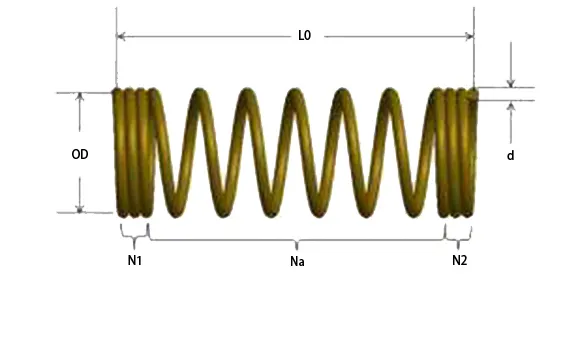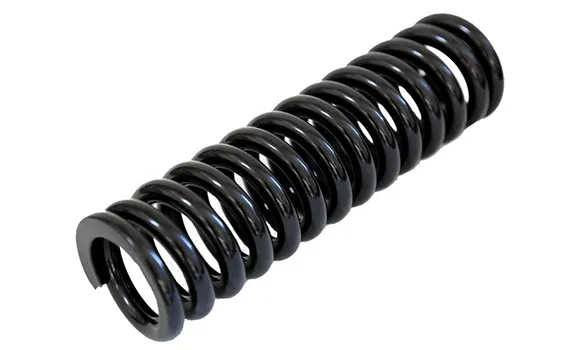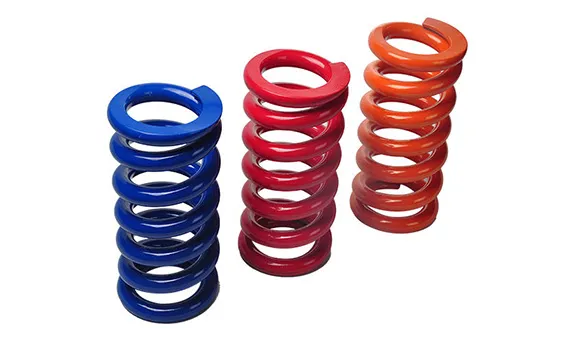# How to Choose a Compression Spring - Design / Measure a Compression Spring

A compression spring is an open-coil helical spring that offers resistance to a compressive force applied axially. They are usually coiled at a constant diameter, though they can be coiled in other needed forms such as conical, concave (barrel), convex (hourglass), or various combinations of these. Here we just total about how to choose a constant dieameter compression springs.As the above picture shows, we can measure a compression spring with the dimensions as followings,

d, the wire diameter

OD, the outside diameter of springs

ID, the inner diameter of springs, which equals to (OD-d)/2

Dm，the mean diameter of springs, which equals to OD-d or ID+d

L0, free length

N1, the closed coils at end one

N2, the closed coils at end two

Na, active coils

N, total coils, which equals to N1+N2+Na

t, pitch, it means the distance between two active coilsAfter measured the dimensions, the paramter of springs can be caculate. The followings is mainly parameters,

Spirngs constant(P', sometime use K), it equals to G*d*d*d*d/(8*Na*Dm*Dm*Dm), this parameter is very important for a compression springs.

Springs index(C), it equals to (OD-d)/d, and it normally should between 4 to 20.

Helical angles(α), it equals to Atg(t/π/Dm), and it should between 4-9°.

Deflection, it means the length that pressed by load.

Max. deflction, the deflction when all coils closed.

Closed Length(Lc), also called solid length, it means the length of when alll the coils closed.

Max. Load, it means the load at the length of max. deflection.

Would you understand of it? If any question still, please contact me, we are spring manufacturer and have more than 60 years experiences, and I have worked with our engieer more than ten years. let's talk more about it, and I will help you to design it.

Request a Quote

We will response your request in 24 hours.